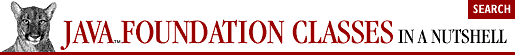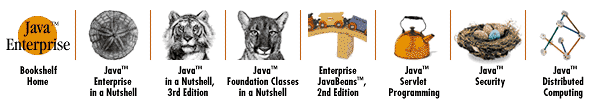home | O'Reilly's CD bookshelfs | FreeBSD | Linux | Cisco | Cisco Exam## 4.3. The Coordinate System

By default, Java 2D uses the same coordinate system as AWT. The origin is in the upper-left corner of the drawing surface. X coordinate values increase to the right, and Y coordinate values increase as they go down. When drawing to a screen or an off-screen image, X and Y coordinates are measured in pixels. When drawing to a printer or other high-resolution device, however, X and Y coordinates are measured in points instead of pixels (and there are 72 points in one inch).

It is instructive to consider in more detail how Java 2D draws to a high-resolution device like a printer. The Java 2D drawing commands you issue express coordinates on the printer paper in units of points. This coordinate system is referred to as "user space." However, different printers print at different resolutions and support different coordinate systems, so when drawing to a device like this, Java 2D must convert your user-space coordinates into printer-specific, device-space coordinates.

On a high-resolution printer, one point in user space may translate into 10 or more pixels in the printer's device space. In order to take full advantage of all this resolution, you need to be able to use coordinates like 75.3 in user space. This brings us to one of the big differences between the Java 2D coordinate system and the AWT system: Java 2D allows coordinates to be expressed as floating-point numbers, instead of restricting them to integers. Throughout the Java 2D API, you'll see methods that accept float values instead of int values.

The distinction between user space and device space is valid even when we are just drawing to the relatively low resolution screen. By default, when drawing to a screen or image, user space is the same as device space. However, the Graphics2D class defines methods that allow you to trivially modify the default coordinate system. For example, you can move the origin of the coordinate system with the translate() method. The following code draws two identical lines at identical positions. The first line is drawn in the default coordinate system, while the second is drawn after calling translate():

```Graphics2D g;                    // Assume this is already initialized
g.drawLine(100, 100, 200, 200);  // Draw in the default coordinate system
g.translate(100.0, 100.0);       // Move the origin down and to the right
g.drawLine(0, 0, 100, 100);      // Draw the same line relative to new origin```

The translate() method is not all that interesting, and, in fact, a version of it existed even before Java 2D. The Graphics2D class also defines scale(), rotate(), and shear() methods that perform more powerful transformations of the coordinate system.

By default, when drawing to the screen, one unit in user space corresponds to one pixel in device space. The scale() method changes this. If you scale the coordinate system by a factor of 10, one unit of user space corresponds to 10 pixels in device space. Note that you can scale by different amounts in the X and Y dimensions. The following code draws the same simple line from 100, 100 to 200, 200 (using the default origin):

```g.scale(2.0, 2.0);
g.drawLine(50, 50, 100, 100);```

You can combine transformations. For example, suppose you are drawing into a 500-pixel-by-500-pixel window and you want to have the origin at the bottom left of the window, with Y coordinates increasing as they go up, rather than as they go down. You can achieve this with two simple method calls:

```g.translate(0.0, 500.0);  // Move the origin to the lower left
g.scale(1.0, -1.0);       // Flip the sign of the coordinate system```

rotate() is another powerful coordinate system transformation method. You specify an angle in radians, and the method rotates the coordinate system by that amount. The direction of rotation is such that points on the positive X axis are rotated in the direction of the positive Y axis. Although you typically do not want to leave your coordinate system in a permanently rotated state, the rotate() method is useful for drawing rotated text or other rotated graphics. For example:

```g.rotate(Math.PI/4);                     // Rotate 45 degrees
g.drawString("Hello world", 300, 300)    // Draw text in this rotated position
g.rotate(-Math.PI/4);                    // Rotate back to normal```

Note that these calls to rotate() rotate the coordinate system about the origin. There is also a three-argument version of the method that rotates about a specified point, which can often be more useful.

The final transformation method defined by Graphics2D is shear(). The effects of this method are not as intuitive as the methods we've already discussed. After a call to shear(), any rectangles you draw appear skewed, as parallelograms.

Any calls you make to translate(), scale(), rotate(), and shear() have a cumulative effect on the mapping from user space to device space. This mapping is encapsulated in a java.awt.geom.AffineTransform object and is one of the graphics attributes maintained by a Graphics2D object. You can obtain a copy of the current transform with getTransform(), and you can set a transform directly with setTransform(). setTransform() is not cumulative. It simply replaces the current user-to-device-space mapping with a new mapping:

```AffineTransform t = g.getTransform(); // Save the current transform
g.rotate(theta);                      // Change the transform
g.drawRect(100, 100, 200, 200);       // Draw something
g.setTransform(t);                    // Restore the transform to its old state```

AffineTransform is used in a number of places in the Java 2D API; we'll discuss it in more detail later in this chapter. Once you understand the details and some of the math behind this class, you can define AffineTransform objects of your own and pass them to setTransform().

Another use of AffineTransform objects is with the transform() method of Graphics2D. This method modifies the current coordinate system, just as translate(), scale(), rotate(), and shear() do. transform() is much more general, however. The AffineTransform object you pass to it can represent any arbitrary combination of translation, scaling, rotation, and shearing.